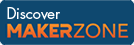Cody

# Problem 189. Sum all integers from 1 to 2^n

Given the number x, y must be the summation of all integers from 1 to 2^x. For instance if x=2 then y must be 1+2+3+4=10.

### Solution Stats

58.3% Correct | 41.7% Incorrect
Last solution submitted on Mar 18, 2019

#### TagsMATLAB and Simulink resources for Arduino, LEGO, and Raspberry Pi